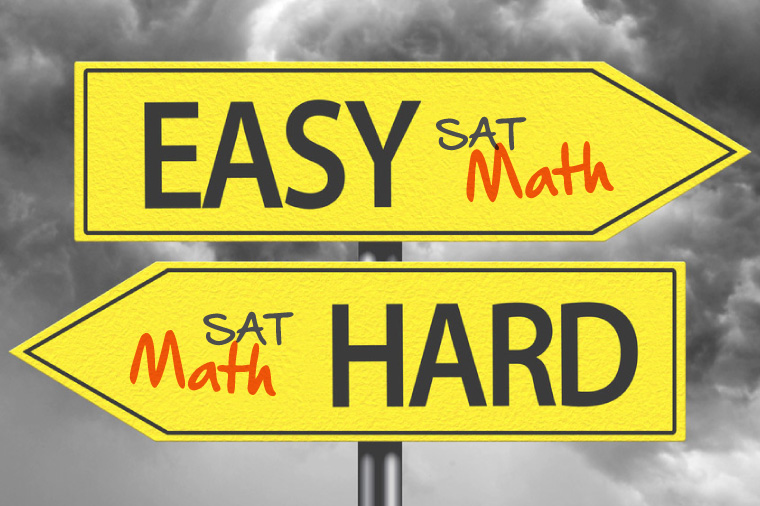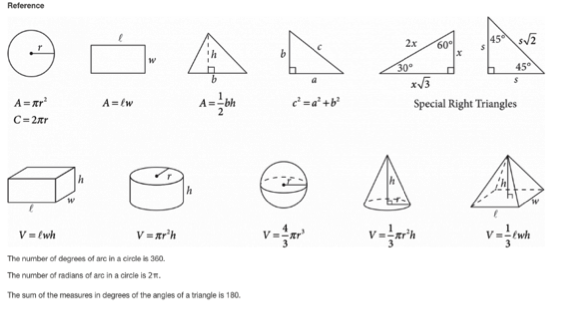## The New SAT Math Easy or Hard?With the release of the new SAT, many students and parents are having a dilemma: the new SAT or the ACT? While changes have certainly been made to the new SAT math section, they do not necessarily make it harder to prepare for. Let’s take a look at things that you should know about the new SAT math.

Topics covered

• Basic algebra (30%): linear equations, systems of linear equations, inequalities
• Advanced topics (60%): ratios, proportions, percentages, units, probabilities, quadratic, exponential and other nonlinear functions
• Geometry (10%): area, volume, lines, angles, triangles, circles, right triangles, the unit circle, trigonometric functions

New SAT math vs. Old SAT math

• Only 4 answer choices, instead of 5:
• Unlike the old SAT test, students now get 4 answer choices instead of 5 on the new test. Additionally, there will not be any penalty for wrong answers. So students don’t have to worry about losing points for wrong guesses.
• The new SAT math has two sections: one with calculator, one without:
• The old SAT math allows you to use a calculator on the entire math section. On the new SAT, you will first spend 55 minutes on the calculator-allowed portion, and then 25 minutes on the non-calculator one. However, the questions in the non-calculator portion should not require complex calculations, but students who are better at mental math will find this portion easier.
• Less focus on geometry:
• On the old SAT, geometry took up about 30% of the math section. On the new SAT, however, geometry only accounts for about 10%. Ironically, the new SAT’s formula reference section provides students with three extra geometry formulae: the volume formulae for spheres, cones, and pyramids.SAT-Math-formulae

• Increased focus on algebra:
• While algebra has always been an integral part of SAT math, it is now even more emphasized due to the reduced focus on geometry. More than half of the questions in the math section will test students’ algebra skills. Some of the algebra questions might appear trickier to some students, as they will test problem solving and analytical skills. For example, the students could be expected to come up with linear equations or systems of linear equations to solve problems, or the student could be asked to analyze or graph non-linear functions to solve other problems.
• Increased focus on modeling:
• This essentially means that the new SAT wants to test how well you can understand equations and variables in a particular context. The questions will require you to analyze the meaning of the number or variable in the equation, and then apply your analysis to solve the problem.
• Addition of trigonometry and imaginary number(i):
• Historically, questions on trigonometry and imaginary numbers had only been a part of the SAT2 Math subject test, until now. Questions involving trigonometry will account for about 5% of all math questions. As for imaginary number, it is sufficient to be familiar with the idea of i=√-1

The new SAT math allows for more time per question than the ACT math

• On the ACT math, students have to answer 60 questions in 60 minutes, giving an average of 1 minute per question. On the new SAT math, students have to answer 38 questions in 55 minutes for the calculator portion, and 20 questions in 25 minutes for the non-calculator portion, which gives an average of 1 minute and 20 seconds per question. The new SAT math definitely allows more time per question than its ACT counterpart. This extra 20 seconds could be what it takes to improve one’s math performance on a college entrance exam.

These changes to the SAT certainly mean that students have to prepare for the test differently than before. If a student were to take the new SAT, algebra should be a major part of his study plan. After all, the questions on the new SAT math are generally more straightforward than those on its predecessor.

Finally, Success Prep is always here to help you succeed in the new SAT. In preparation for the December 2016 new SAT test, Success Prep offers classes starting in October and continuing through November at either Morehouse College or Decatur High School in Atlanta, GA. Additionally, Success Prep offers online self-study tools, such as practice questions, solution videos, tips, etc. For more information, check out Success Prep SAT Prep Class – Atlanta GA.